Updating search results...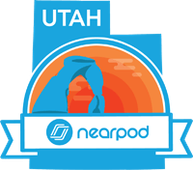# Nearpod Math Lessons

This collection includes Nearpod lessons that support teaching math standards.

12 affiliated resources

# Search Resources

View
Selected filters:Rating
0.0 stars

In this lesson students will use geogebra and other tools to discover how to calculate the slope of a line. They will also recognize lines that have 0 and undefined slope.

Subject:
Mathematics
Secondary Mathematics
Material Type:
Activity/Lab
Game
Interactive
Author:
Mikki Stuart
07/12/2022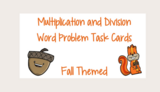Unrestricted Use
Public Domain
Rating
0.0 stars

Here is a Nearpod that was created to give students another opportunity to solve real world problems using a Fall theme. These problems consist of word problems with basic multiplication and division strategies and facts. A fun new way to learn math.

Subject:
Elementary Mathematics
Mathematics
Material Type:
Homework/Assignment
Author:
Katelyn Gustafson
12/22/2021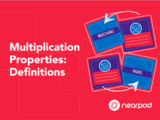Only Sharing Permitted
CC BY-NC-ND
Rating
0.0 stars

In this math skill-based practice activity, students strengthen their understanding of associative, commutative, and distributive properties. They complete a vocabulary challenge with definitions.

Subject:
Elementary Mathematics
Mathematics
Material Type:
Nearpod
Author:
Nearpod Team
07/12/2022Only Sharing Permitted
CC BY-NC-ND
Rating
0.0 stars

Length times width equals… In this funny Nearpod lesson, students learn how to calculate the area of rectangles. Students will learn how to calculate area by counting un and then by using a formula.

Subject:
Elementary Mathematics
Mathematics
Material Type:
Nearpod
Author:
Nearpod
07/28/2022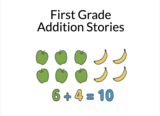Rating
0.0 stars

This Nearpod activity takes 45-60 minutes. Students will learn how to draw pictures for addition story problems. They will learn about a plus and equal symbol. They will also be introduced to creating addition number sentences.

Subject:
Elementary Mathematics
Mathematics
Material Type:
Interactive
Author:
Malaree Parry
12/29/2022Some Rights Reserved
Rating
0.0 stars

This is a Nearpod Time to Climb with Fluency Practice within 20.

Subject:
Elementary Mathematics
Mathematics
Material Type:
Activity/Lab
Author:
Jenifer Palomin
10/20/2021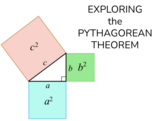Unrestricted Use
Public Domain
Rating
0.0 stars

This is an Interactive Nearpod that introduces students to the Pythagorean Theorem. It helps students identify the legs and the hypotenuse of a right triangle. It also helps students understand how to find the length of the missing side.

Subject:
Mathematics
Secondary Mathematics
Material Type:
Activity/Lab
Author:
mathantics.com
06/29/2021Rating
0.0 stars

Easy to follow the discussion about the need for fractions and how we use them in real life. A short video about fractions including the components of fractions and a brief description of how we can learn more about them and why. D

Subject:
Elementary Mathematics
Mathematics
Material Type:
Nearpod
Author:
jMontserrat" Julieta Ulansosky Ketsy Garcia/ Rebekah Bergman
02/22/2022Rating
0.0 stars

On this nearpod students can review Standard 1.NBT.2 Understand that the two digits of a two-digit number represent amounts of tens and ones. Understand the following as special cases:

Subject:
Mathematics
Material Type:
Nearpod
Author:
Nearpod Team
07/28/2022Rating
0.0 stars

This is a Nearpod lesson.
In this warm-up lesson students practice identifying the place value and value of digits. They also write numbers. This lesson is most appropriate for 3rd grade students. The lesson takes approximately 20 minutes of class time to complete. By the end of this warm-up lesson, students will be able to read numbers in expanded and word form using place value. They will also be able to write numbers using place value and identify the place value of digits in numbers. This warm-up lesson features work in pairs to help students identify place value. Working with a partner, students identify place values and write numbers. Students review different numbers and demonstrate their understanding of digits in different place values. They read a description of a number based on its place values, and they write the corresponding number. Students also look at the expanded form, as well as the word form, and write the number on the line. The lesson includes a quiz on place value in which students must demonstrate their fluency with expanded form, word form, numerical form, and place value. (Description written by Nearpod)

Subject:
Elementary Mathematics
Mathematics
Material Type:
Lesson
Author:
Nearpod
10/01/2020Only Sharing Permitted
CC BY-NC-ND
Rating
0.0 stars

In this Nearpod lesson on fractions, students will review fractions and learn how to identify and create equivalent fractions.

Subject:
Elementary Mathematics
Mathematics
Material Type:
Nearpod
Author:
Nearpod
08/02/2021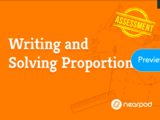Only Sharing Permitted
CC BY-NC-ND
Rating
0.0 stars

This is a 10 slide interactive lesson that will help students learn and practice writing and solving proportions.

Subject:
Mathematics
Secondary Mathematics
Material Type:
Lesson
Author:
Nearpod